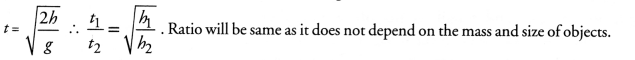## NCERT Exemplar Solutions for Class 9 Science Chapter 10 Gravitation

These Solutions are part of NCERT Exemplar Solutions for Class 9 Science . Here we have given NCERT Exemplar Solutions for Class 9 Science Chapter 10 Gravitation

MULTIPLE CHOICE QUESTIONS

Question 1.
Two objects of different masses falling freely near the surface of moon would
(a) have same velocities at any instant
(b) have different accelerations
(c) experience forces of same magnitude
(d) undergo a change in their inertia.
(a) Explanation : During free fall, acceleration remains the same irrespective of the mass of the object. Force is directly proportional to the mass of a freely falling object.

More Resources

Question 2.
The value of acceleration due to gravity
(a) is same on equator and poles
(b) is least on poles
(c) is least on equator
(d) increases from pole to equator.
(c) Explanation :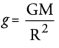Question 3.
The gravitational force between two objects is F. If masses of both objects are halved without changing distance between them, then the gravitation force would become
(a) F/4
(b) F/2
(c) F
(d) 2FQuestion 4.
A boy is whirling a stone tied with a string in an horizontal circular path the string breaks, the stone
(a) will continue to move in the circular path
(b) will move along a straight line towards the centre of the circular path
(c) will move along a straight line tangential to the circular path
(d) will move along a straight line perpendicular to the circular path away from the boy.
(c) Explanation : Due to inertia of directions.

Question 5.
In the relation F = G M m/d, the quantity G
(a) depends on the value of g at the place of observation
(b) is used only when the earth is one of the two masses
(c) is greatest at the surface of the earth
(d) is universal constant of nature.
(d) Explanation : The value of ‘G’ is same throughout the universe.

Question 6.
Law of gravitation gives the gravitational force between
(a) the earth and a point mass only
(b) the earth and sun only
(c) any two bodies having some mass
(d) two charged bodies only.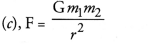Question 7.
The value of quantity G in the law of gravitation
(a) depends on mass of earth only
(b) depends on radius of earth only
(c) depends on both mass and radius of earth
(d) is independent of mass and radius of the earth.
(d) Explanation : G is universal constant.

Question 8.
Two particles are placed at some distance. If the mass of each of the two particles is doubled, keeping the distance between them unchanged, the value of gravitational force between them will be
(a) 1/4 times
(b) 4 times
(c) 1/2 times
(d) unchanged.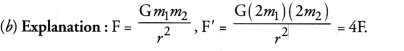Question 9.
The atmosphere is held to the earth by
(a) gravity
(b) wind
(c) clouds
(d) earths magnetic field.
(a).

Question 10.
The force of attraction between two unit point masses separated by a unit distance is called
(a) gravitational potential
(b) acceleration due to gravity
(c) gravitational field
(d) universal gravitational constant.Question 11.
The weight of an object at the centre of the earth of radius R is
(a) zero
(b) infinite
(c) R times the weight at the surface of the earth
(d) 1/R2 times the weight at surface of the earth.
(a) Explanation : W = mg. The value of ‘g’ at the centre of earth is zero.

Question 12.
An apple falls from a tree because of gravitational attraction between the earth and apple. If F1 is the magnitude of force exerted by the earth on the apple and F2 is the magnitude of force exerted by apple on earth, then
(a) F1 is very much greater than F2
(b) F2 is very much greater than F1
(c) F1 is only a little greater than F2
(d) F1 and F2 are equal.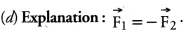Question 13.
What is the source of centripetal force that a planet requires to revolve around the sun ? On what factors does that force depend ?
The source of centripetal force that a planet requires to revolve around the sun is the gravitational force between the sun and the planet. Thus,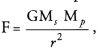where m is the mass of the sun, is the mass of the planet and r is the distance between the sun and the planet.
Thus, the force depends upon

1. the mass of the sun,
2. the mass of the planet and
3. the distance between the sun and the planet.

Question 14.
On the earth, a stone is thrown from a height in a direction parallel to the earths surface while another stone is simultaneously dropped from the same height. Which stone would reach the ground first and why ?
Both stones will reach the ground simultaneously. Initial velocity of both the stones in the downward direction is zero and the acceleration of both the stones in the downward direction is same and equal to g.so both stones take same time to reach the ground.

Question 15.
Suppose gravity of earth suddenly becomes zero, then in which direction will the moon begin to move if no other celestial body affects it ?
Gravity of earth provides necessary centripetal force to the moon to move in a circular path around the earth. If gravity becomes zero, there is no centripetal force and hence, the moon will begin to move in a straight line along to the tangent at the point on the circular path due to inertia of direction.

Question 16.
Identical packets are dropped from two aeroplanes. One above the equator and the other above the north pole both at height h. Assuming all conditions are identical will those packets take same time to reach the surface of earth. Justify your answer.
(CBSE Sample Paper)
Time taken by an object to fall through height h at a place is given by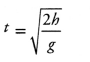Since, value of ‘g’ at poles is greater than at the equator, therefore, packet dropped above the north pole will take less time than the packet dropped above the equator to reach the surface of the earth.

Question 17.
The weight of any person on the moon is about 1/6 times that on the earth. He can lift a mass of 15 kg on the earth. Whatwill be the maximum mass, which can be lifted by the same force applied by the person on the moon ? (CBSE Sample Paper)Question 18.
Calculate the average density of earth in terms of g, G,m?Question 19.
The earth is acted upon by gravitation of sun, even though it does not fall into the sun. Why ? (CBSE 2012)
The earth revolves around the sun. The centripetal force is needed by the earth to revolve around the sun. This centripetal force is provided by the gravitational force between the sun and the earth. The earth keeps on moving around the sun as long as gravitational force between the earth and the sun acts on it.

Question 20.
How does the weight of an object vary with respect to mass and radius of the earth. In a hypothetical case, if the diameter of the earth becomes half of its present value and its mass becomes four times of its present value, then how would the weight of any object on the surface of the earth be affected ? (CBSE 2012)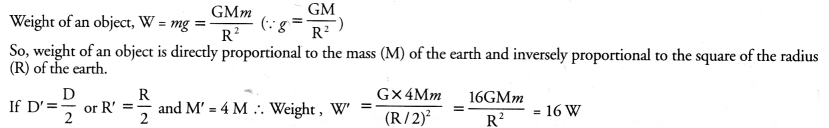Question 21.
How does the force of attraction between two bodies depend upon their masses and distance between them ? A student thought that two bricks tied together would fall faster than a single one under the action of gravity. Do you agree with this hypothesis or not ? Comment.
The force of attraction between two bodies of masses m1 and m2 and separated by a distance r is given byThis force is known as gravitational force. The gravitational force is

1. directly proportional to the product of the masses of two bodies and
2. inversely proportional to the square of the distance between them.

The hypothesis is not correct. This is because, all bodies in the absence of any force of friction fall with the same acceleration (known as acceleration due to gravity) irrespective of their masses. Hence two bricks tied together will not fall faster than a single brick under the action of gravity.

Question 22.
Two objects of masses m1 and mhaving the same size are dropped simultaneously from heights h1 and h2– respectively. Find out the ratio of time they would take in reaching the ground. Will this ratio remain the same if

1. one of the object is hollow and the other one is solid and
2. both of them are hollow, size remaining the same in each case. Give reason.